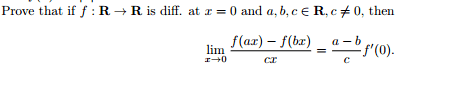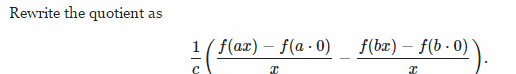# Calculus Limit Proof

## Homework Statement

I am posting this for another student who I noticed did not have the proof in the problem. Here is what she said. Lets try and help her out.

I have been working on the problem below and I am stuck. I am stuck primarily because of the part where is says x=0. If x-0, it should cancel everything out. The derivative of 0 is 0 so will cancel everything out I think, so I am not sure if that is the reasoning and the proof behind it.## The Attempt at a Solution

She said:

I thought I should start this way, but I am not 100% sure.[/B]

stevendaryl
Staff Emeritus
The meaning of "$f(x)$ is differentiable at $x=0$" is that near $x=0$, we can write:
$\dfrac{f(x) - f(0)}{x} = f'(0) + \epsilon(x)$
where $\epsilon(x)$ is a correction term that goes to zero as $x \rightarrow 0$. So we can rewrite $f(x)$ as:
$f(x) = f(0) + x (f'(0) + \epsilon(x))$
So try using that expression to rewrite $f(ax)$ and $f(bx)$.